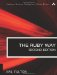# Section 5.30. Conclusion

### 5.29. Caching Functions with memoize

Suppose you have a computationally expensive mathematical function that will be called repeatedly in the course of execution. If speed is critical and you can afford to sacrifice a little memory, it may be effective to store the function results in a table and look them up. This makes the implicit assumption that the function will likely be called more than once with the same parameters; we are simply avoiding "throwing away" an expensive calculation only to redo it later on. This technique is sometimes called memoizing, hence the name of the memoize library.

This is not part of the standard library, so you'll have to install it.

The following example defines a complex function called zeta. (This solves a simple problem in population genetics, but we won't explain it here.)

`require 'memoize' include Memoize def zeta(x,y,z)   lim = 0.0001   gen = 0   loop do     gen += 1     p,q = x + y/2.0, z + y/2.0     x1, y1, z1 =  p*p*1.0, 2*p*q*1.0, q*q*0.9     sum = x1 + y1 + z1     x1 /= sum     y1 /= sum     z1 /= sum     delta = [[x1,x],[y1,y],[z1,z]]     break if delta.all? {|a,b| (a-b).abs < lim }     x,y,z = x1,y1,z1   end       gen end g1 = zeta(0.8,0.1,0.1) memoize(:zeta)            # store table in memory g2 = zeta(0.8,0.1,0.1) memoize(:zeta,"z.cache")  # store table on disk g3 = zeta(0.8,0.1,0.1)`

Notice that we can specify a filename if we want. This may slow us down a little, but it saves memory and allows us to persist the memoization over subsequent program executions.

In our informal tests, we ran each of these 50,000 times in a tight loop. We found that g2 was calculated about 1,100 times faster than g1, and g3 about 700 times faster. Your results will vary.

One more thing should be noted here. The memoize library is not just for mathematical functions. It can be used for any computationally expensive method.The Ruby Way, Second Edition: Solutions and Techniques in Ruby Programming (2nd Edition)
ISBN: 0672328844
EAN: 2147483647
Year: 2004
Pages: 269
Authors: Hal Fulton

Similar book on Amazon

flylib.com © 2008-2017.
If you may any questions please contact us: flylib@qtcs.net